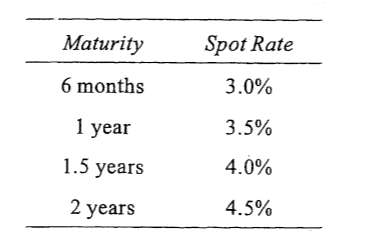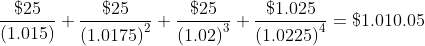Scan QR code or get instant email to install app

Question:

# Consider the following Treasury spot rates expressed as bond equivalent yields:If a Treasury note with two years remaining to maturity has a 5% semiannual coupon and is priced at \$1,008, the note is:A underpriced.
explanation

1212The market value of the Treasury note is the present value of the remaining coupons plus the present value of the principal, discounted at the semiannual rates available from dividing each annual spot rate in the table by two.
value of T-note =At 1,008, the T-note is priced below the present value of its cash flows (1,010) and is therefore underpriced.